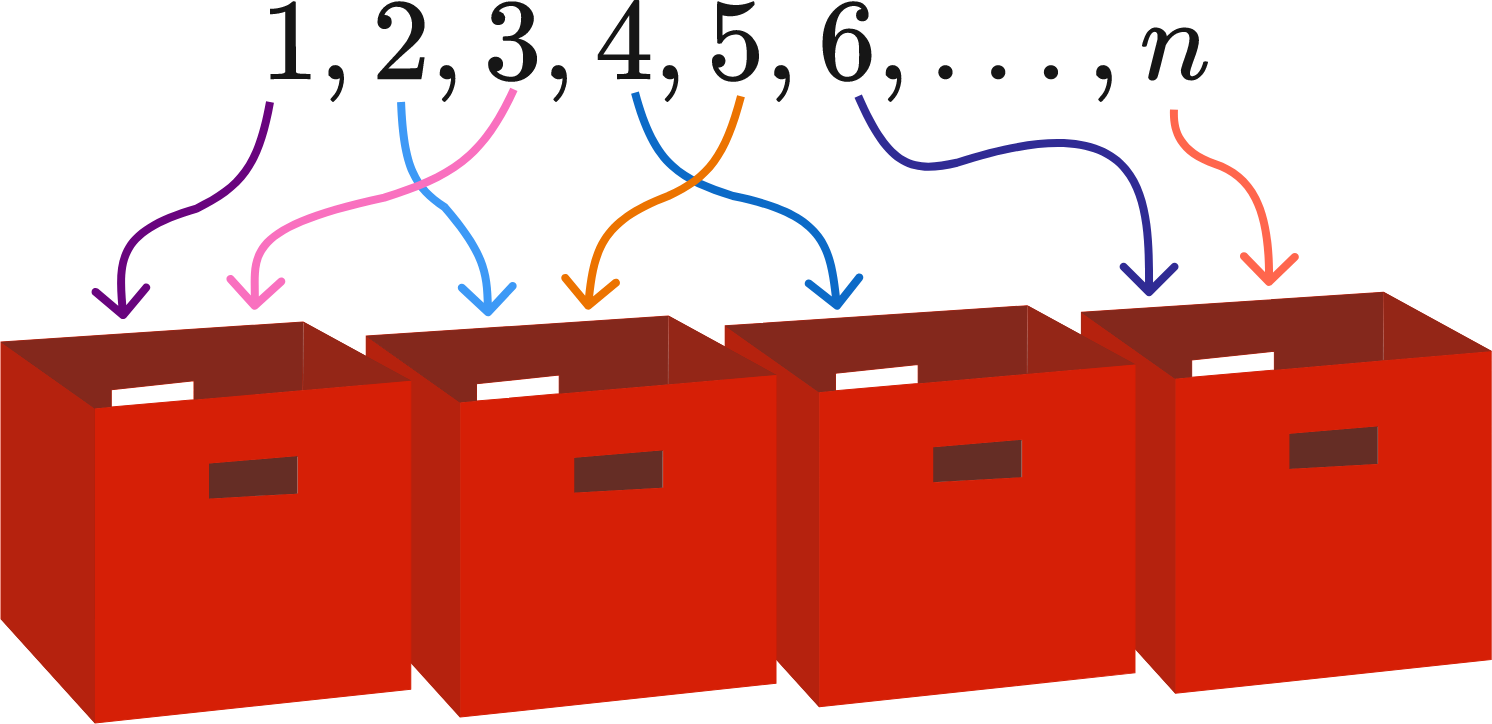# 4 square bins

Probability Level 2The integers 1 through $n$ (inclusive) are separated into four bins.

For each bin, no two different numbers in it can multiply together to form a square number.

What is the largest $n$ for which this is possible?

If you think the answer is infinite, please put 99999 as your answer.

×

Problem Loading...

Note Loading...

Set Loading...